# NCERT Exemplar Class 11 Physics Solutions for Chapter 11 - Thermal Properties Of Matter

NCERT Exemplar Class 11 Physics Chapter 11 Thermal properties of matter is an important study resource for the students who are preparing seriously for CBSE Class 11 Physics, engineering and medical entrance examination.

NCERT Exemplar Class 11 Physics Chapter 11 pdf presents you varieties of questions like MCQs, short answer questions, thermal properties of matter NEET questions, fill in the blanks, match the following, worksheets and numerical problems. Students are advised to get tuned in with this study material that can help you in taking thermal properties of matter notes.

The Thermal properties of matter describe the effect heat has on different states of matter. It is a physical property of matter and the temperature helps to define the state of the matter. Thermal conductivity and Thermal diffusivity are some of the different thermal properties of matter. Some other topics that we can learn in this chapter are:

1. Thermal Expansion
2. Heat Transfer
3. Calorimetry
4. Temperature and Heat
5. Specific Heat Capacity

Know more about NCERT Exemplar Class 11 Physics Chapter 11 Thermal properties of matter from the PDF below:

## Download PDF of NCERT Exemplar Solutions for Class 11 Physics Chapter 11 Thermal properties of matter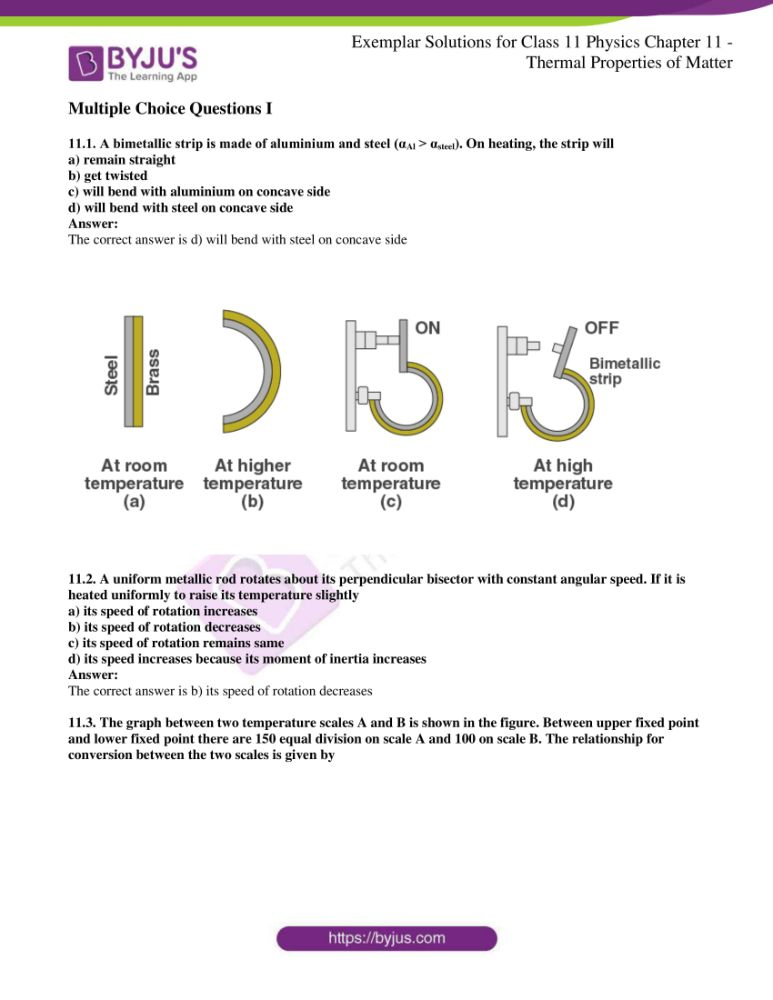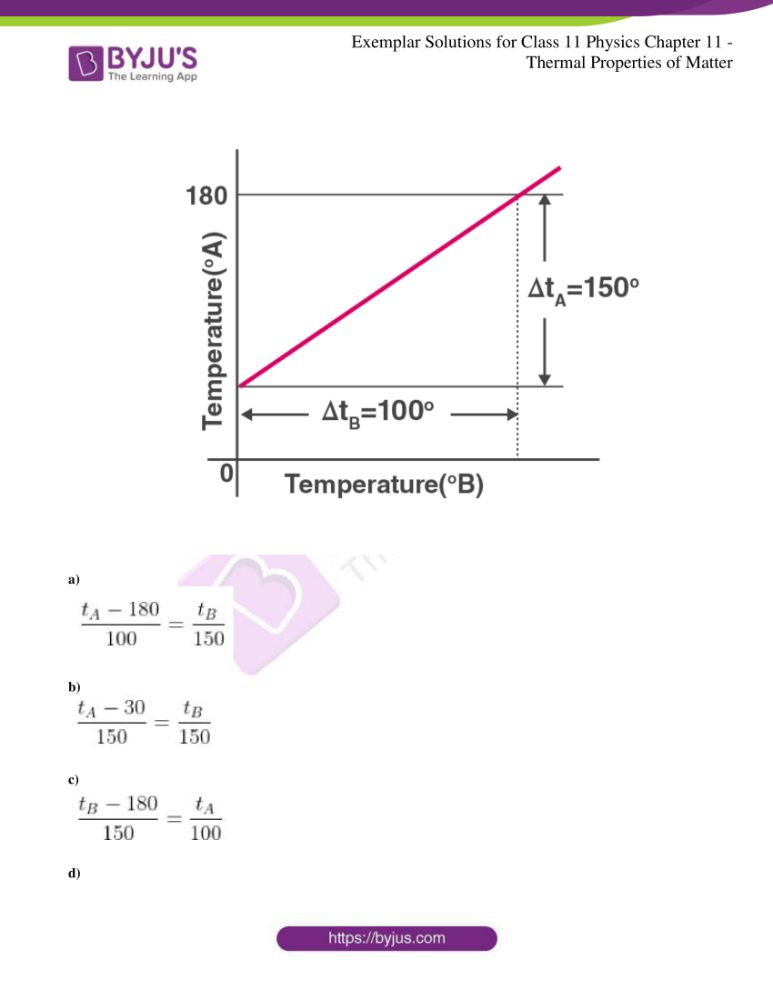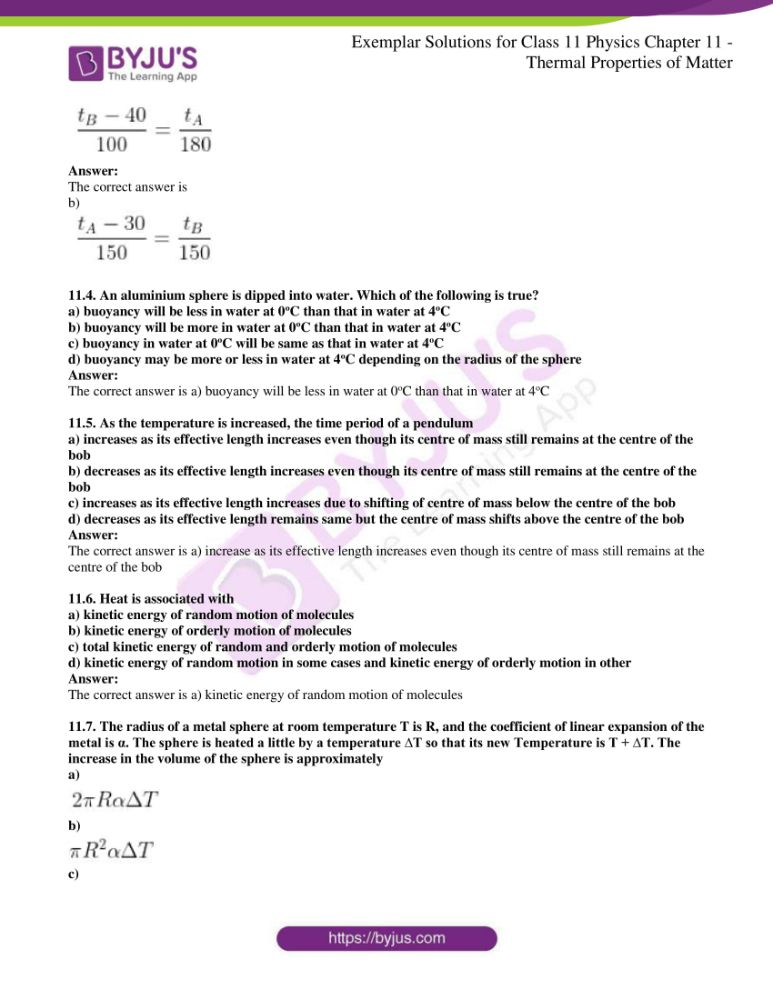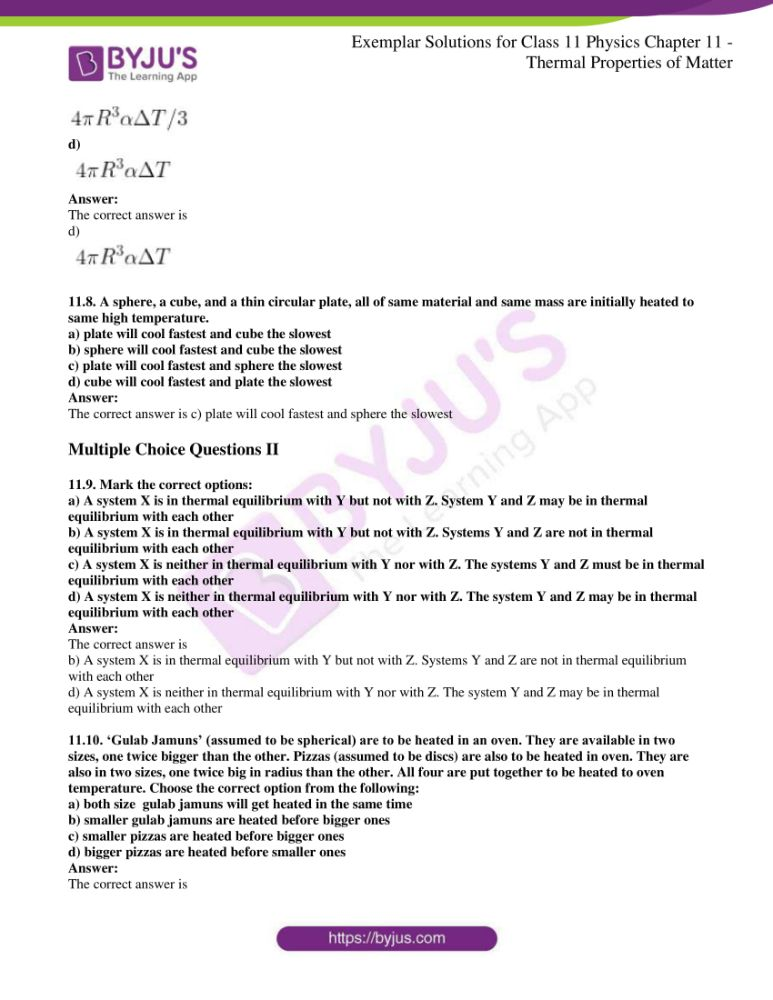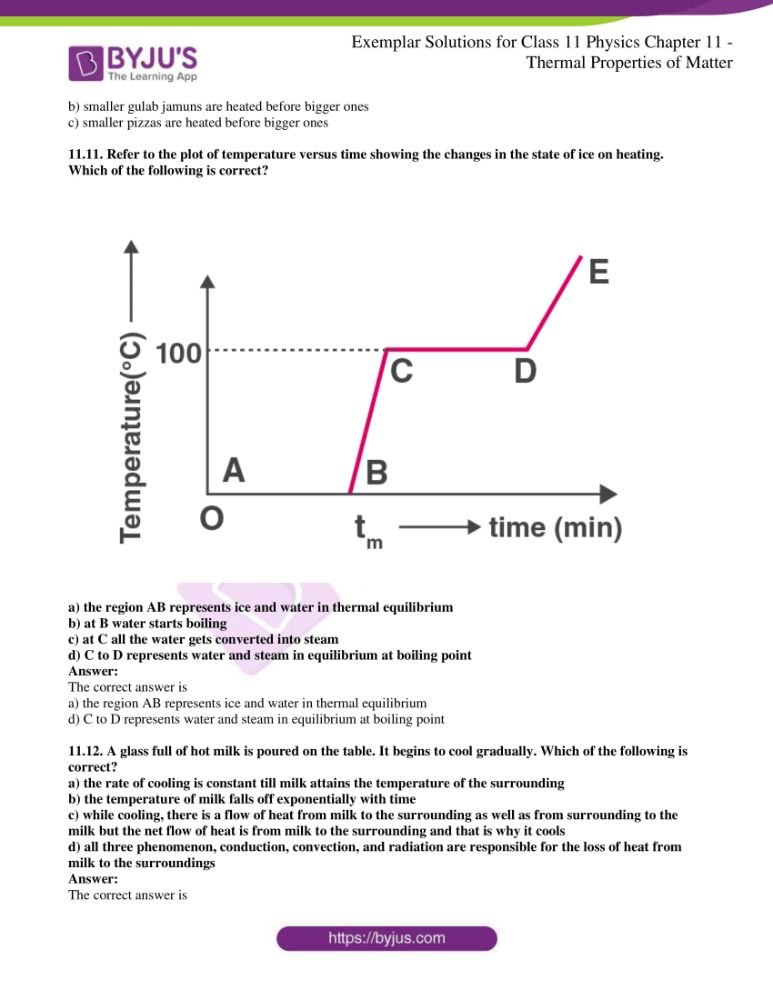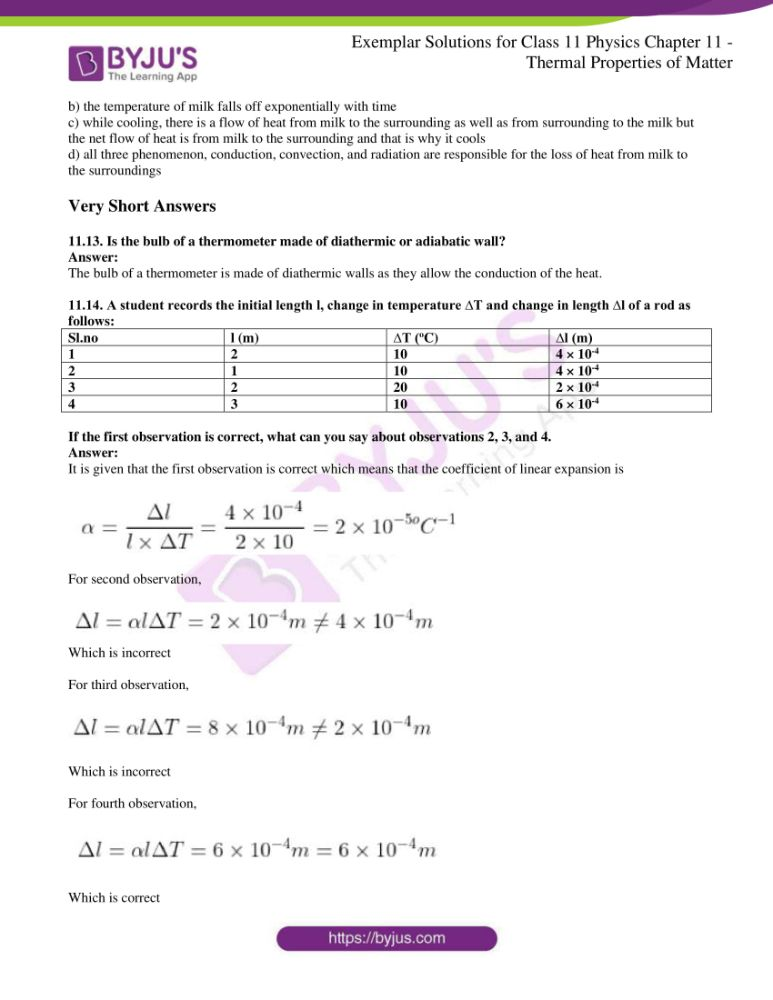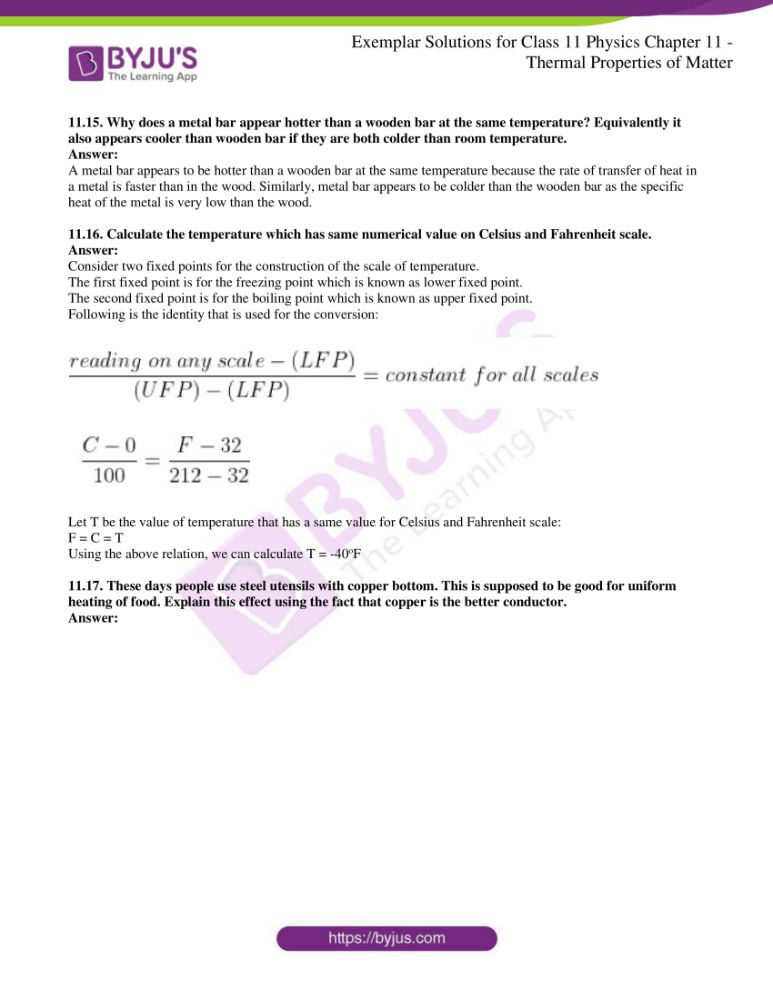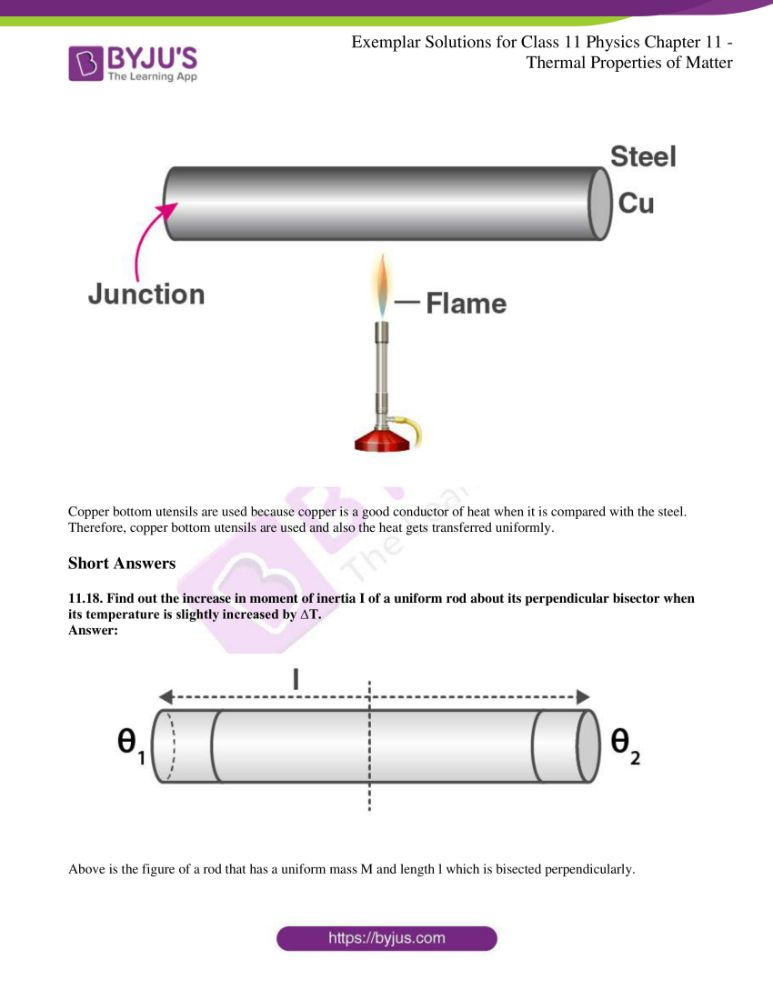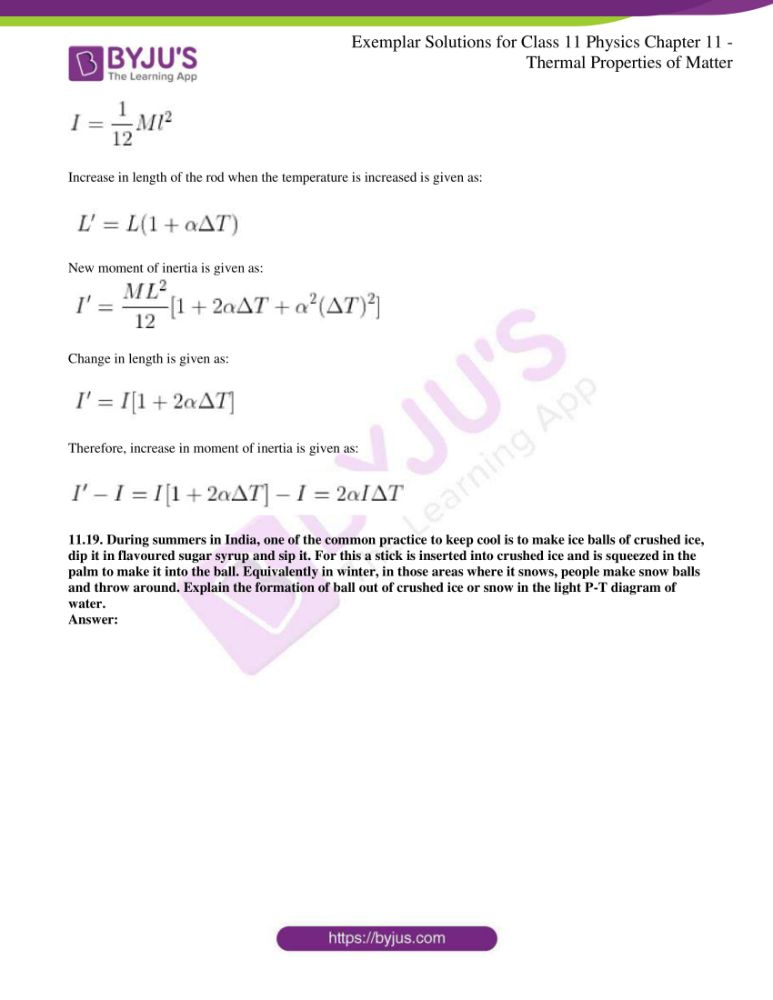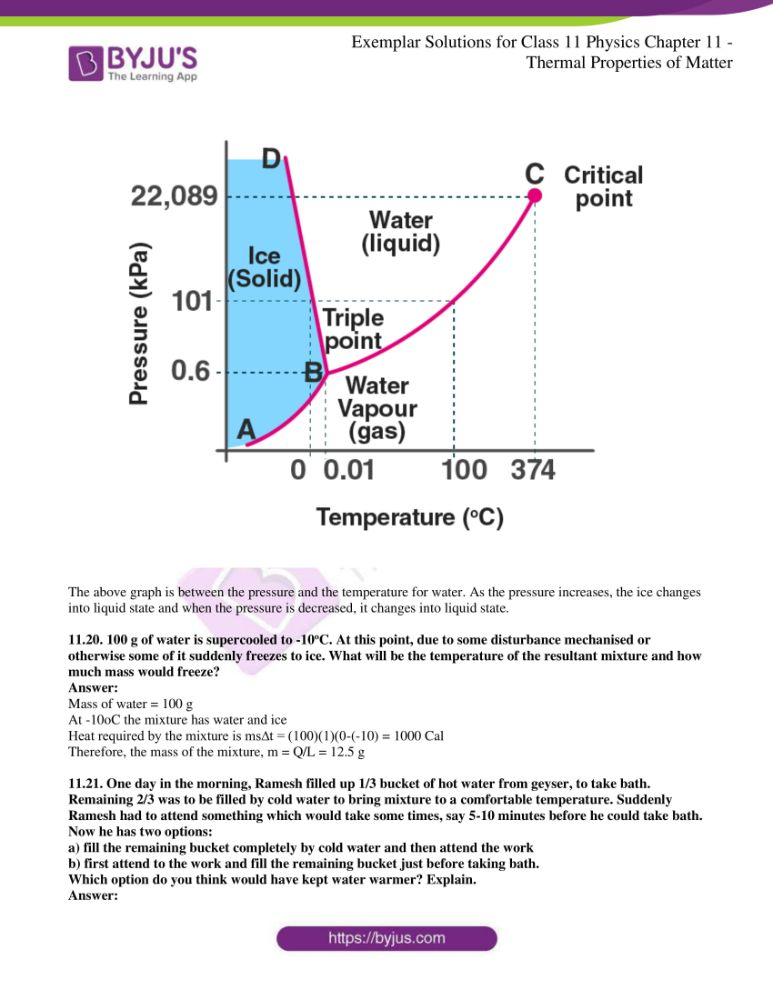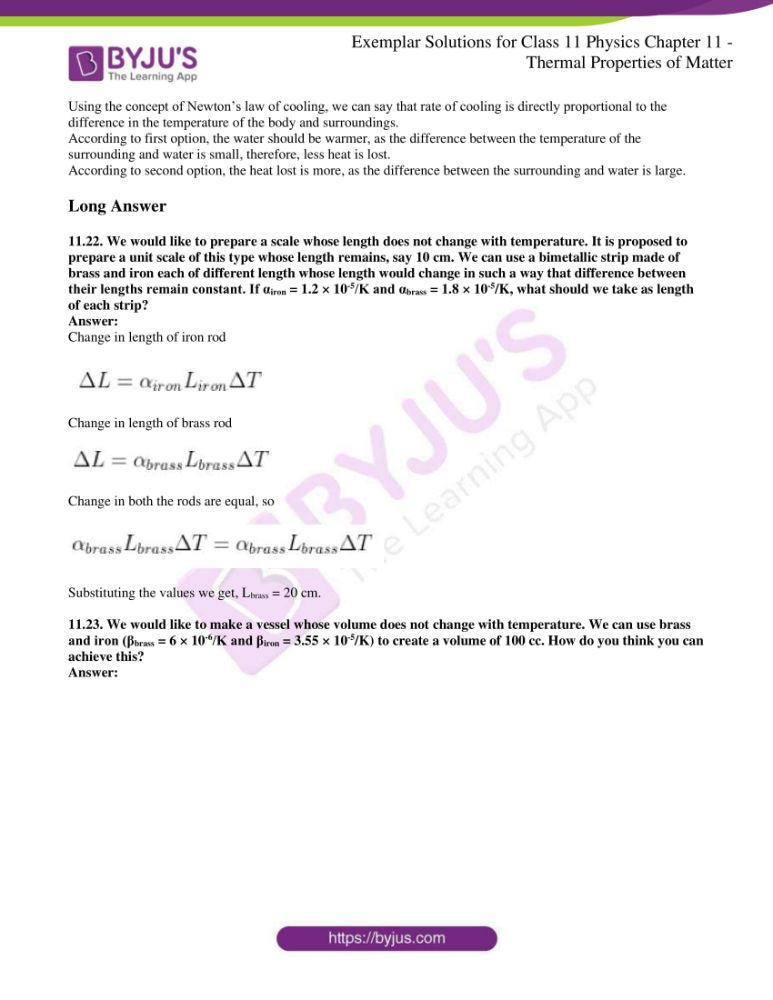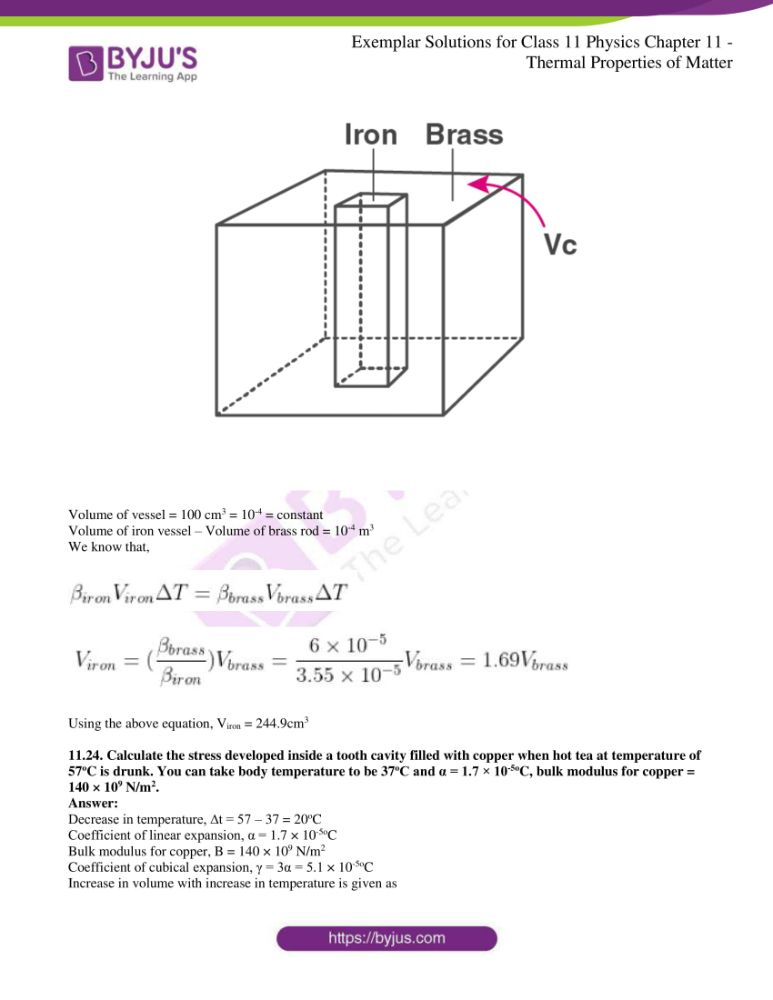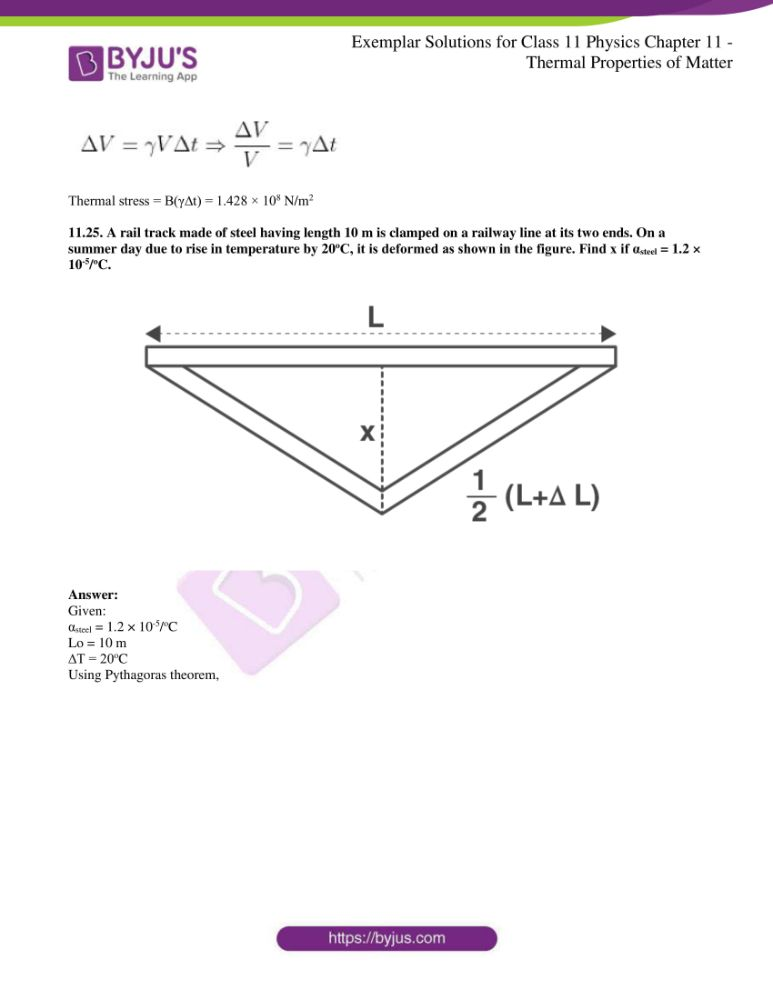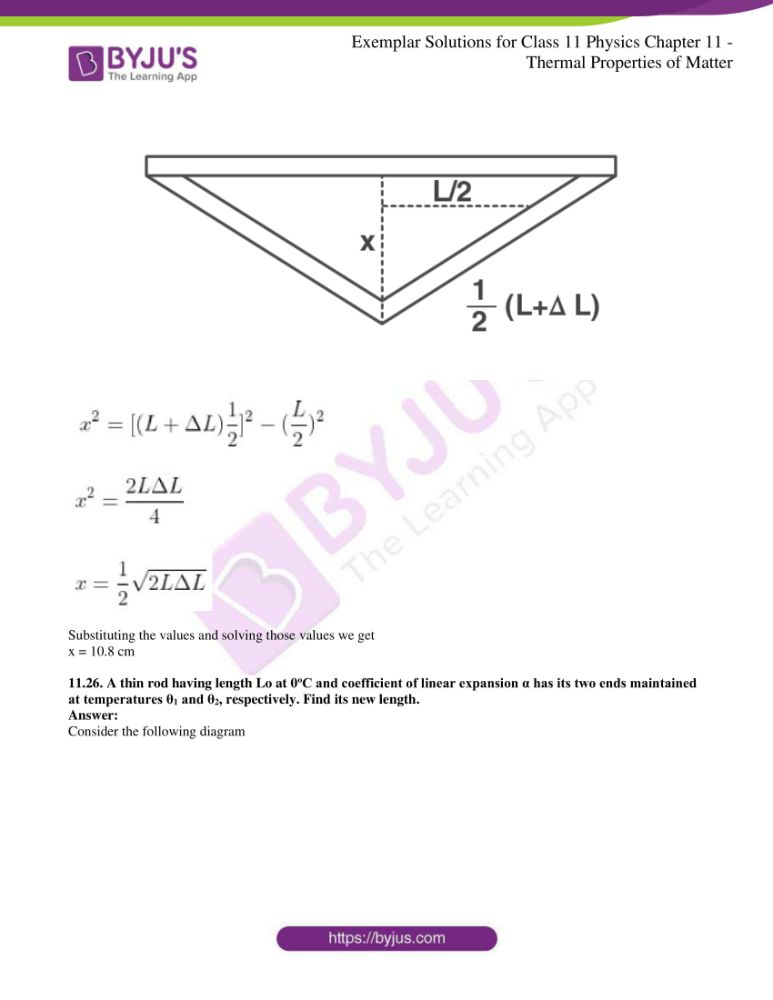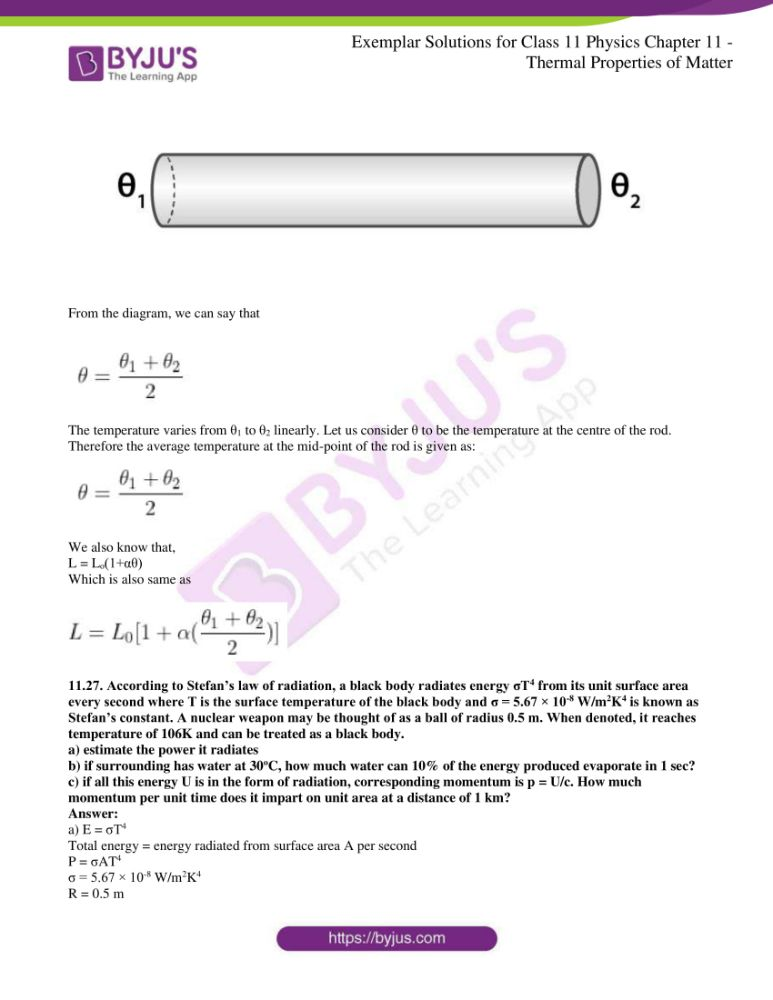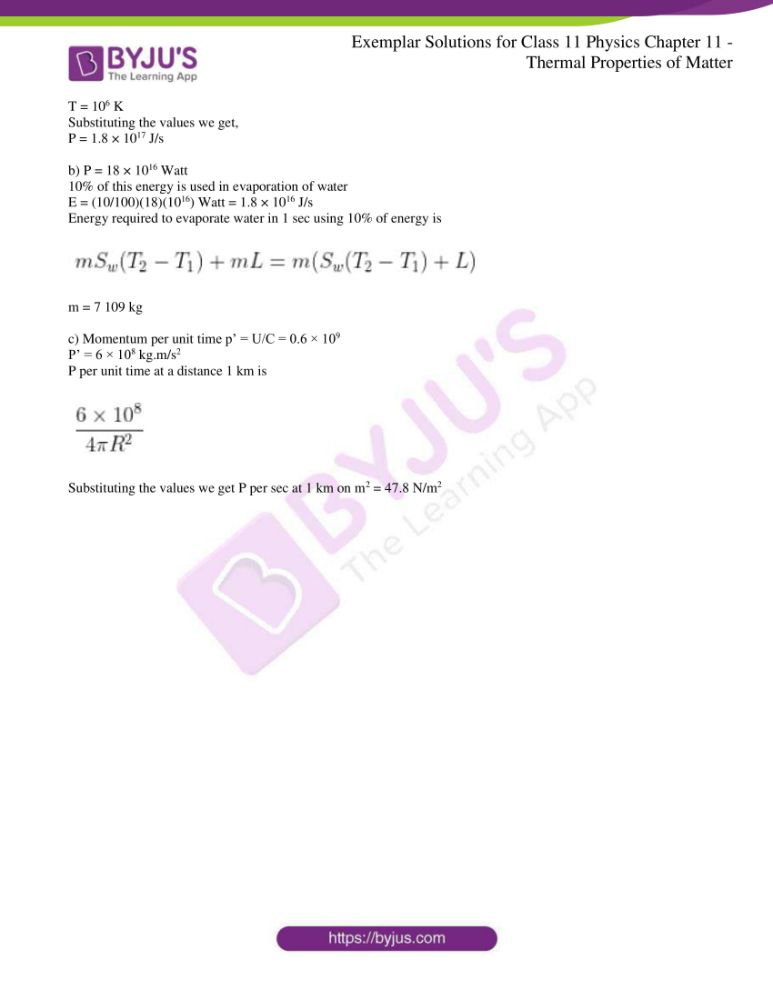### Multiple Choice Questions I

11.1. A bimetallic strip is made of aluminium and steel (αAl > αsteel). On heating, the strip will

a) remain straight

b) get twisted

c) will bend with aluminium on the concave side

d) will bend with steel on the concave side

The correct answer is d) will bend with steel on the concave side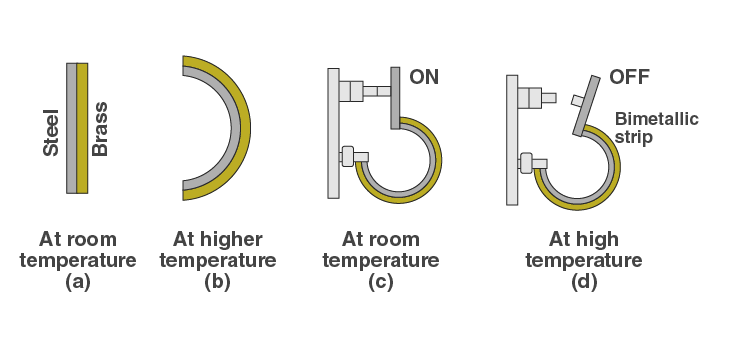11.2. A uniform metallic rod rotates about its perpendicular bisector with constant angular speed. If it is heated uniformly to raise its temperature slightly

a) its speed of rotation increases

b) its speed of rotation decreases

c) its speed of rotation remains the same

d) its speed increases because its moment of inertia increases

The correct answer is b) its speed of rotation decreases

11.3. The graph between two temperature scales A and B is shown in the figure. Between upper fixed point and the lower fixed point, there are 150 equal division on scale A and 100 on scale B. The relationship for conversion between the two scales is given by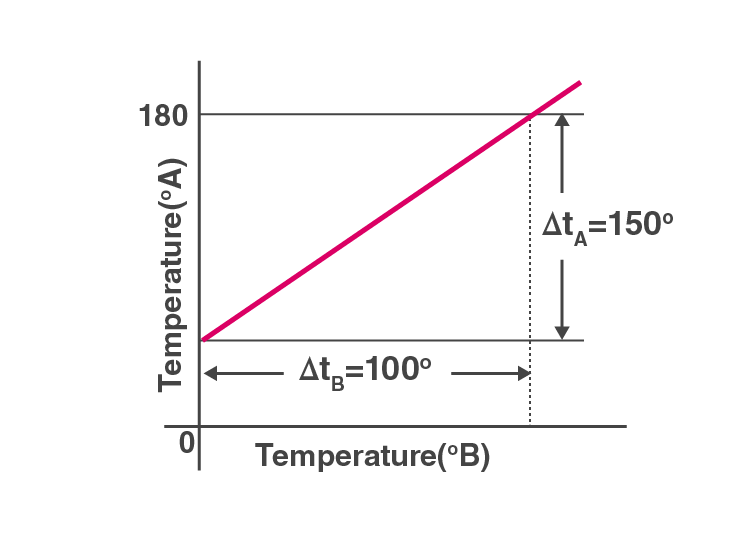a)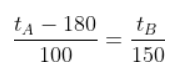b)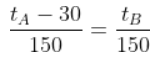c)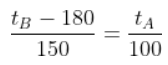d)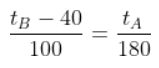b)11.4. An aluminium sphere is dipped into water. Which of the following is true?

a) buoyancy will be less in water at 0oC than that in water at 4oC

b) buoyancy will be more in the water at 0oC than that in water at 4oC

c) buoyancy in water at 0oC will be same as that in the water at 4oC

d) buoyancy may be more or less in water at 4oC depending on the radius of the sphere

The correct answer is a) buoyancy will be less in water at 0oC than that in water at 4oC

11.5. As the temperature is increased, the time period of a pendulum

a) increases as its effective length increases even though its centre of mass still remains at the centre of the bob

b) decreases as its effective length increases even though its centre of mass still remains at the centre of the bob

c) increases as its effective length increases due to shifting of the centre of mass below the centre of the bob

d) decreases as its effective length remains the same but the centre of mass shifts above the centre of the bob

The correct answer is a) increase as its effective length increases even though its centre of mass still remains at the centre of the bob

11.6. Heat is associated with

a) kinetic energy of random motion of molecules

b) kinetic energy of orderly motion of molecules

c) total kinetic energy of random and orderly motion of molecules

d) kinetic energy of random motion in some cases and kinetic energy of orderly motion in other

The correct answer is a) kinetic energy of random motion of molecules

11.7. The radius of a metal sphere at room temperature T is R, and the coefficient of linear expansion of the metal is α. The sphere is heated a little by a temperature ∆T so that its new Temperature is T + ∆T. The increase in the volume of the sphere is approximately

a)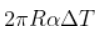b)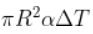c)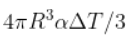d)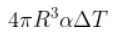d)11.8. A sphere, a cube, and a thin circular plate, all of the same material and same mass are initially heated to same high temperature.

a) plate will cool fastest and cube the slowest

b) sphere will cool fastest and cube the slowest

c) plate will cool fastest and sphere the slowest

d) cube will cool fastest and plate the slowest

The correct answer is c) plate will cool fastest and sphere the slowest

### Multiple Choice Questions II

11.9. Mark the correct options:

a) A system X is in thermal equilibrium with Y but not with Z. System Y and Z may be in thermal equilibrium with each other

b) A system X is in thermal equilibrium with Y but not with Z. Systems Y and Z are not in thermal equilibrium with each other

c) A system X is neither in thermal equilibrium with Y nor with Z. The systems Y and Z must be in thermal equilibrium with each other

d) A system X is neither in thermal equilibrium with Y nor with Z. The system Y and Z may be in thermal equilibrium with each other

b) A system X is in thermal equilibrium with Y but not with Z. Systems Y and Z are not in thermal equilibrium with each other

d) A system X is neither in thermal equilibrium with Y nor with Z. The system Y and Z may be in thermal equilibrium with each other

11.10. ‘Gulab Jamuns’ (assumed to be spherical) are to be heated in an oven. They are available in two sizes, one twice bigger than the other. Pizzas (assumed to be discs) are also to be heated in the oven. They are also in two sizes, one twice big in radius than the other. All four are put together to be heated to oven temperature. Choose the correct option from the following:

a) both size gulab jamuns will get heated at the same time

b) smaller gulab jamuns are heated before bigger ones

c) smaller pizzas are heated before bigger ones

d) bigger pizzas are heated before smaller ones

b) smaller gulab jamuns are heated before bigger ones

c) smaller pizzas are heated before bigger ones

11.11. Refer to the plot of temperature versus time showing the changes in the state of ice on heating. Which of the following is correct?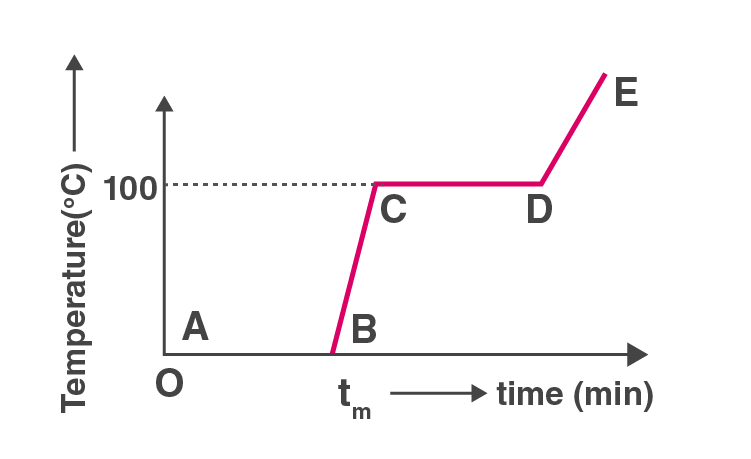a) the region AB represents ice and water in thermal equilibrium

b) at B water starts boiling

c) at C all the water gets converted into steam

d) C to D represents water and steam in equilibrium at boiling point

a) the region AB represents ice and water in thermal equilibrium

d) C to D represents water and steam in equilibrium at boiling point

11.12. A glass full of hot milk is poured on the table. It begins to cool gradually. Which of the following is correct?

a) the rate of cooling is constant till milk attains the temperature of the surrounding

b) the temperature of milk falls off exponentially with time

c) while cooling, there is a flow of heat from milk to the surrounding as well as from surrounding to the milk but the net flow of heat is from milk to the surrounding and that is why it cools

d) all three phenomena, conduction, convection, and radiation are responsible for the loss of heat from milk to the surroundings

b) the temperature of milk falls off exponentially with time

c) while cooling, there is a flow of heat from milk to the surrounding as well as from surrounding to the milk but the net flow of heat is from milk to the surrounding and that is why it cools

d) all three phenomena, conduction, convection, and radiation are responsible for the loss of heat from milk to the surroundings

11.13. Is the bulb of a thermometer made of diathermic or adiabatic wall?

The bulb of a thermometer is made of diathermic walls as they allow the conduction of the heat.

11.14. A student records the initial length l, change in temperature ∆T and change in length ∆l of a rod as follows:

 Sl.no l (m) ∆T (oC) ∆l (m) 1 2 10 4 × 10-4 2 1 10 4 × 10-4 3 2 20 2 × 10-4 4 3 10 6 × 10-4

If the first observation is correct, what can you say about observations 2, 3, and 4.

It is given that the first observation is correct which means that the coefficient of linear expansion is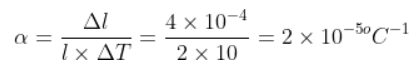For second observation,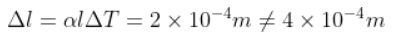Which is incorrect

For third observation,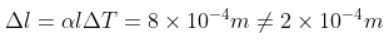Which is incorrect

For fourth observation,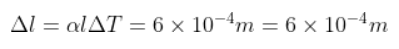Which is correct

11.15. Why does a metal bar appear hotter than a wooden bar at the same temperature? Equivalently it also appears cooler than wooden bar if they are both colder than room temperature.

A metal bar appears to be hotter than a wooden bar at the same temperature because the rate of transfer of heat in a metal is faster than in the wood. Similarly, metal bar appears to be colder than the wooden bar as the specific heat of the metal is very low than the wood.

11.16. Calculate the temperature which has same numerical value on Celsius and Fahrenheit scale.

Consider two fixed points for the construction of the scale of temperature.

The first fixed point is for the freezing point which is known as lower fixed point.

The second fixed point is for the boiling point which is known as upper fixed point.

Following is the identity that is used for the conversion: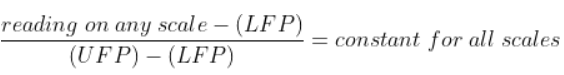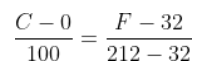Let T be the value of temperature that has a same value for Celsius and Fahrenheit scale:

F = C = T

Using the above relation, we can calculate T = -40oF

11.17. These days people use steel utensils with copper bottom. This is supposed to be good for uniform heating of food. Explain this effect using the fact that copper is the better conductor.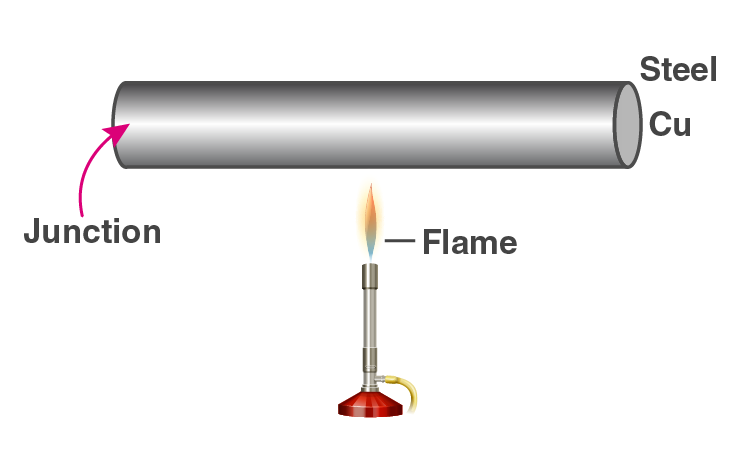Copper bottom utensils are used because copper is a good conductor of heat when it is compared with the steel. Therefore, copper bottom utensils are used and also the heat gets transferred uniformly.

11.18. Find out the increase in moment of inertia I of a uniform rod about its perpendicular bisector when its temperature is slightly increased by ∆T.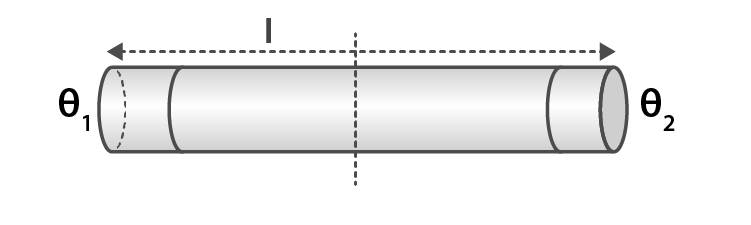Above is the figure of a rod that has a uniform mass M and length l which is bisected perpendicularly.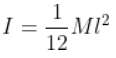Increase in length of the rod when the temperature is increased is given as: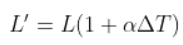New moment of inertia is given as: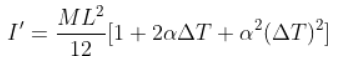Change in length is given as: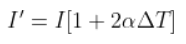Therefore, increase in moment of inertia is given as: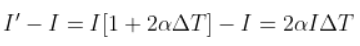11.19. During summers in India, one of the common practice to keep cool is to make ice balls of crushed ice, dip it in flavoured sugar syrup and sip it. For this a stick is inserted into crushed ice and is squeezed in the palm to make it into the ball. Equivalently in winter, in those areas where it snows, people make snow balls and throw around. Explain the formation of ball out of crushed ice or snow in the light P-T diagram of water.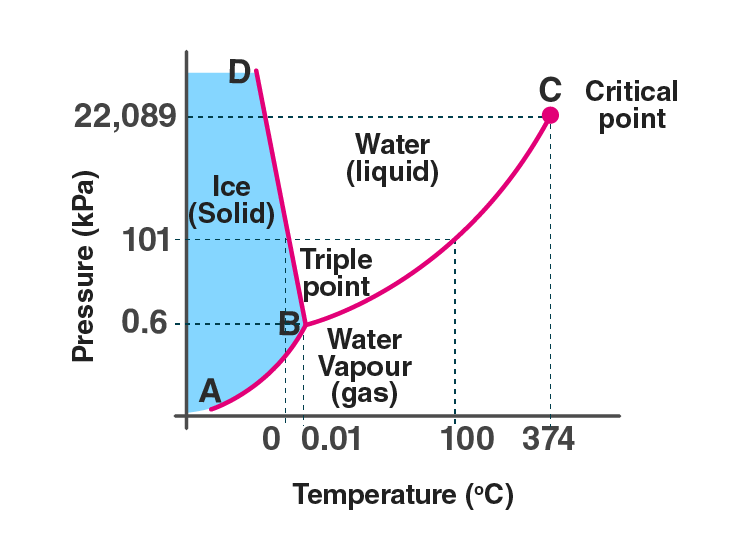The above graph is between the pressure and the temperature for water. As the pressure increases, the ice changes into liquid state and when the pressure is decreased, it changes into liquid state.

11.20. 100 g of water is supercooled to -10oC. At this point, due to some disturbance mechanised or otherwise some of it suddenly freezes to ice. What will be the temperature of the resultant mixture and how much mass would freeze?

Mass of water = 100 g

At -10oC the mixture has water and ice

Heat required by the mixture is ms∆t = (100)(1)(0-(-10) = 1000 Cal

Therefore, the mass of the mixture, m = Q/L = 12.5 g

11.21. One day in the morning, Ramesh filled up 1/3 bucket of hot water from geyser, to take bath. Remaining 2/3 was to be filled by cold water to bring mixture to a comfortable temperature. Suddenly Ramesh had to attend something which would take some times, say 5-10 minutes before he could take bath. Now he has two options:

a) fill the remaining bucket completely by cold water and then attend the work

b) first attend to the work and fill the remaining bucket just before taking bath.

Which option do you think would have kept water warmer? Explain.

Using the concept of Newton’s law of cooling, we can say that rate of cooling is directly proportional to the difference in the temperature of the body and surroundings.

According to first option, the water should be warmer, as the difference between the temperature of the surrounding and water is small, therefore, less heat is lost.

According to second option, the heat lost is more, as the difference between the surrounding and water is large.

11.22. We would like to prepare a scale whose length does not change with temperature. It is proposed to prepare a unit scale of this type whose length remains, say 10 cm. We can use a bimetallic strip made of brass and iron each of different length whose length would change in such a way that difference between their lengths remain constant. If αiron = 1.2 × 10-5/K and αbrass = 1.8 × 10-5/K, what should we take as length of each strip?

Change in length of iron rod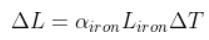Change in length of brass rod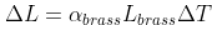Change in both the rods are equal, so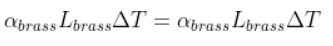Substituting the values we get, Lbrass = 20 cm.

11.23. We would like to make a vessel whose volume does not change with temperature. We can use brass and iron (βbrass = 6 × 10-6/K and βiron = 3.55 × 10-5/K) to create a volume of 100 cc. How do you think you can achieve this?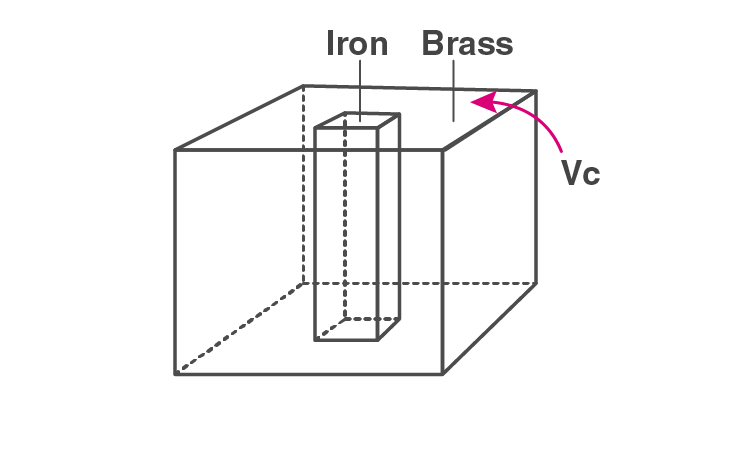Volume of vessel = 100 cm3 = 10-4 = constant

Volume of iron vessel – Volume of brass rod = 10-4 m3

We know that,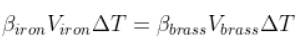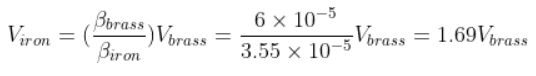Using the above equation, Viron = 244.9cm3

11.24. Calculate the stress developed inside a tooth cavity filled with copper when hot tea at temperature of 57oC is drunk. You can take body temperature to be 37oC and α = 1.7 × 10-5oC, bulk modulus for copper = 140 × 109 N/m2.

Decrease in temperature, ∆t = 57 – 37 = 20oC

Coefficient of linear expansion, α = 1.7 × 10-5oC

Bulk modulus for copper, B = 140 × 109 N/m2

Coefficient of cubical expansion, γ = 3α = 5.1 × 10-5oC

Increase in volume with increase in temperature is given as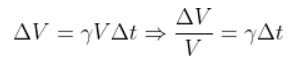Thermal stress = B(γ∆t) = 1.428 × 108 N/m2

11.25. A rail track made of steel having length 10 m is clamped on a railway line at its two ends. On a summer day due to rise in temperature by 20oC, it is deformed as shown in the figure. Find x if αsteel = 1.2 × 10-5/oC.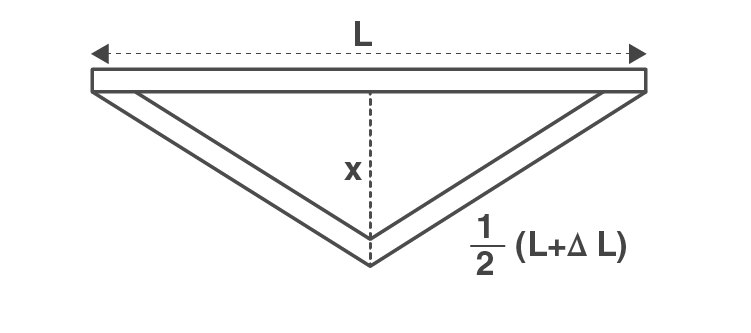Given:

αsteel = 1.2 × 10-5/oC

Lo = 10 m

∆T = 20oC

Using Pythagoras theorem,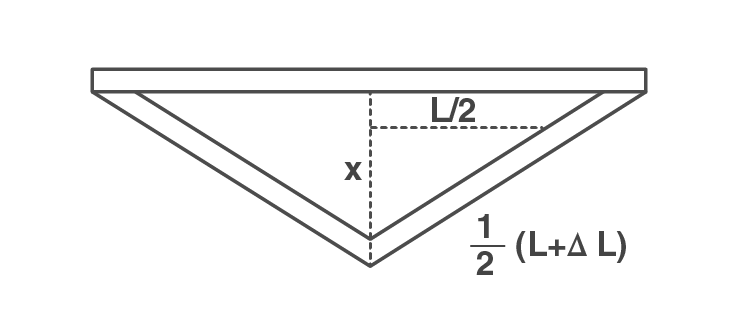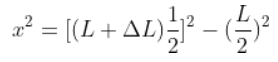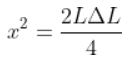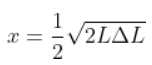Substituting the values and solving those values we get

x = 10.8 cm

11.26. A thin rod having length Lo at 0oC and coefficient of linear expansion α has its two ends maintained at temperatures θ1 and θ2, respectively. Find its new length.

Consider the following diagram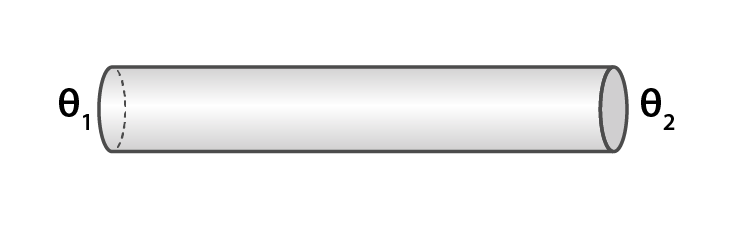From the diagram, we can say that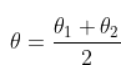The temperature varies from θ1 to θ2 linearly. Let us consider θ to be the temperature at the centre of the rod. Therefore the average temperature at the mid-point of the rod is given as:We also know that,

L = Lo(1+αθ)

Which is also same as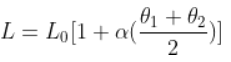11.27. According to Stefan’s law of radiation, a black body radiates energy σT4 from its unit surface area every second where T is the surface temperature of the black body and σ = 5.67 × 10-8 W/m2K4 is known as Stefan’s constant. A nuclear weapon may be thought of as a ball of radius 0.5 m. When denoted, it reaches temperature of 106K and can be treated as a black body.

a) estimate the power it radiates

b) if surrounding has water at 30oC, how much water can 10% of the energy produced evaporate in 1 sec?

c) if all this energy U is in the form of radiation, corresponding momentum is p = U/c. How much momentum per unit time does it impart on unit area at a distance of 1 km?

a) E = σT4

Total energy = energy radiated from surface area A per second

P = σAT4

σ = 5.67 × 10-8 W/m2K4

R = 0.5 m

T = 106 K

Substituting the values we get,

P = 1.8 × 1017 J/s

b) P = 18 × 1016 Watt

10% of this energy is used in evaporation of water

E = (10/100)(18)(1016) Watt = 1.8 × 1016 J/s

Energy required to evaporate water in 1 sec using 10% of energy is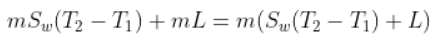m = 7 109 kg

c) Momentum per unit time p’ = U/C = 0.6 × 109

P’ = 6 × 108 kg.m/s2

P per unit time at a distance 1 km is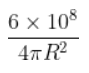Substituting the values we get P per sec at 1 km on m2 = 47.8 N/m2

### Why Opt for BYJU’S?

CBSE Class 11 is an important stage in students life. Concepts studied in Class 11 will help the students throughout their coming future. It is essential for students to get well versed on the basic concepts involved in Class 11 in order to avoid difficulty in future.

In order to help students, BYJU’S brings innovative learning opportunities that can help the students not only in understanding the topic but also assist them in memorising the concepts for a very long period of time.